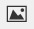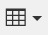PSABM6M5 - Student Facing Task---Acc7.3 Lesson 4 Reasoning about Equations and Tape Diagrams (Part 1) (6.EE.B.5, 7.EE.B.3, 7.EE.B.4.a)
Part A)

Find a solution to each equation without writing anything down.x = _____

Type your answer below as a number (example: 5, 3.1, 4 1/2, or 3/2):
Part B)x = _____

Type your answer below as a number (example: 5, 3.1, 4 1/2, or 3/2):
Part C)x = _____

Type your answer below as a number (example: 5, 3.1, 4 1/2, or 3/2):
Part D)x = _____

Type your answer below as a number (example: 5, 3.1, 4 1/2, or 3/2):
Part A)

Draw a tape diagram to represent each situation. For some of the situations, you need to decide what to represent with a variable.

Diego has 7 packs of markers. Each pack has x markers in it. After Lin gives him 9 more markers, he has a total of 30 markers.

Draw your diagram on a piece of paper, take a picture of the diagram, and upload it using the image upload button.  Alternatively, you can make a tape diagram using the table buttonPart B)

Elena is cutting a 30-foot piece of ribbon for a craft project. She cuts off 7 feet, and then cuts the remaining piece into 9 equal lengths of x feet each.

Draw your diagram on a piece of paper, take a picture of the diagram, and upload it using the image upload button.  Alternatively, you can make a tape diagram using the table buttonPart C)

A construction manager weighs a bundle of 9 identical bricks and a 7-pound concrete block. The bundle weighs 30 pounds.

Draw your diagram on a piece of paper, take a picture of the diagram, and upload it using the image upload button.  Alternatively, you can make a tape diagram using the table buttonPart D)

A skating rink charges a group rate of \$9 plus a fee to rent each pair of skates. A family rents 7 pairs of skates and pays a total of \$30.

Draw your diagram on a piece of paper, take a picture of the diagram, and upload it using the image upload button.  Alternatively, you can make a tape diagram using the table buttonPart E)

Andre bakes 9 pans of brownies. He donates 7 pans to the school bake sale and keeps the rest to divide equally among his class of 30 students.

Draw your diagram on a piece of paper, take a picture of the diagram, and upload it using the image upload button.  Alternatively, you can make a tape diagram using the table buttonPart F)

Each situation in the previous activity is represented by one of these equations:

•••Match each situation to an equation.

Part G)

7x + 9 = 30

x = _____

Type your answer below as a number (example: 5, 3.1, 4 1/2, or 3/2):
Part H)x = _____

Type your answer below as a number (example: 5, 3.1, 4 1/2, or 3/2):
Part I)x = _____

Type your answer below as a number (example: 5, 3.1, 4 1/2, or 3/2):
Part J)

What does each solution tell you about its situation?